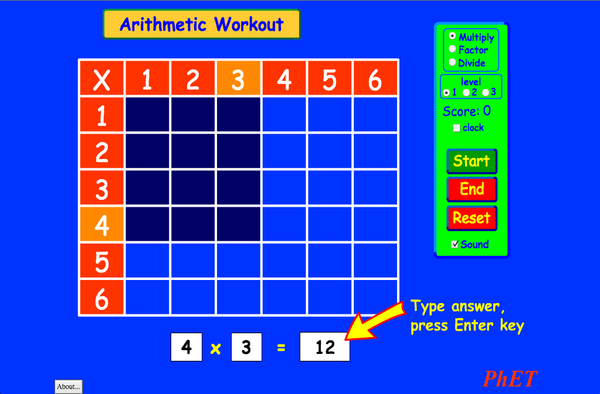# 算术下载嵌入代码 关闭 嵌入模拟程序控制 你可以通过改变嵌入的代码改变程序的宽度和高度 插入一张图片，当点击这个图片时启动仿真程序。回到HTML5 版本

• 增殖

### 示范 学习目标

• 解释乘法表是如何促进乘法、因子以及分解的理解。提高乘法、因子分解、除法的精确度。

### 标准对齐

#### Common Core - Math

3.OA.A.1
Interpret products of whole numbers, e.g., interpret 5 × 7 as the total number of objects in 5 groups of 7 objects each. For example, describe a context in which a total number of objects can be expressed as 5 × 7.
3.OA.A.2
Interpret whole-number quotients of whole numbers, e.g., interpret 56 ÷ 8 as the number of objects in each share when 56 objects are partitioned equally into 8 shares, or as a number of shares when 56 objects are partitioned into equal shares of 8 objects each. For example, describe a context in which a number of shares or a number of groups can be expressed as 56 ÷ 8.
3.OA.A.4
Determine the unknown whole number in a multiplication or division equation relating three whole numbers. For example, determine the unknown number that makes the equation true in each of the equations 8 × ? = 48, 5 = _ ÷ 3, 6 × 6 = ?
3.OA.B.5
Apply properties of operations as strategies to multiply and divide.2 Examples: If 6 × 4 = 24 is known, then 4 × 6 = 24 is also known. (Commutative property of multiplication.) 3 × 5 × 2 can be found by 3 × 5 = 15, then 15 × 2 = 30, or by 5 × 2 = 10, then 3 × 10 = 30. (Associative property of multiplication.) Knowing that 8 × 5 = 40 and 8 × 2 = 16, one can find 8 × 7 as 8 × (5 + 2) = (8 × 5) + (8 × 2) = 40 + 16 = 56. (Distributive property.)
3.OA.B.6
Understand division as an unknown-factor problem. For example, find 32 ÷ 8 by finding the number that makes 32 when multiplied by 8.
3.OA.C.7
Fluently multiply and divide within 100, using strategies such as the relationship between multiplication and division (e.g., knowing that 8 × 5 = 40, one knows 40 ÷ 5 = 8) or properties of operations. By the end of Grade 3, know from memory all products of two one-digit numbers.
3.OA.D.9
Identify arithmetic patterns (including patterns in the addition table or multiplication table), and explain them using properties of operations. For example, observe that 4 times a number is always even, and explain why 4 times a number can be decomposed into two equal addends.

### 教师指南仿真程序控制概述,简化模型,学生视角的思考 ( PDF ).

### 教师提交的活动

Juego de Aritmética (Basado en Indagación)Trish Loeblein, traducido por Diana López 初中

How do PhET simulations fit in my middle school program?Sarah Borenstein 初中 其它 化学

곱셈 나눗셈 연습 SIM 사용설명서 이화국(Wha Kuk Lee) 初中
K-5

ARITHMETIC_DIVISON Arlete Cabral K-5 实验室 数学
OBJETO DE APRENDIZAGEM: ARITHMETIC Arlete Moura de Oliveira Cabral e Maria Cleide da Silva Barroso K-5 指导 数学
Operações Aritméticas Fundamentais Georgyana Cidrão; Francisco Régis Vieira Alves K-5 指导

MS and HS TEK to Sim Alignment Elyse Zimmer 初中

Math facts - Using the Multiplication Chart Tapan Sarkar 初中 实验室 数学
Back to the Basics Nyetta Abernathy 初中

K-5

Discovering divisibility rules Stacy Larson 高中

Windows Macintosh Linux
Microsoft Windows
XP/Vista/7/8.1/10
Macromedia Flash 9 or later
OS X 10.9.5 or later
Macromedia Flash 9 or later
Macromedia Flash 9 or later